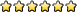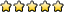## Experimental Design B/C

Jim_RPosts: 283
Joined: May 13th, 2001, 4:22 pm
Has thanked: 0
Been thanked: 0
Contact:

### Experimental Design B/C

-The path of the Administrator is beset on all sides by the inequities of the selfish and the tyranny of evil men.

-Nothing\'s gonna get deleted. We\'re gonna be like three little Fonzies here. And what\'s Fonzie like? Come on, what\'s Fonzie like?
-Cool?
-Correctamundo. And that\'s what we\'re gonna be. We\'re gonna be cool. Now, I\'m gonna count to three, and when I count three, you let go of your mouse, and back away from the keyboard. But when you do it, you do it cool. Ready? One... two... three.
HeavyHitter406
MemberPosts: 45
Joined: April 13th, 2014, 5:50 pm
Division: C
State: IL
Has thanked: 0
Been thanked: 0

### Re: Experimental Design B/C

I'm new to division C...how do you do Standard of Deviation? That is part of the Statistics in C, right?
2014 (Wright State/Lisle/Grayslake/Regionals/State/Nationals)

Water Quality: 8/1/2/1/2/3
Experimental Design: 12/1/5/5/8/37
Wheeled Vehicle: 32/6/6/2/11/5
actionpotential
MemberPosts: 12
Joined: September 25th, 2014, 5:15 pm
Division: C
Has thanked: 0
Been thanked: 0

### Re: Experimental Design B/C

Hey Heavyhitter!

So I've always been confused by that big complicated expression myself. Standard deviation is basically measure of how spread out your data values are.

Analyzed step by step:

1. Take the mean of the data. (Symbol is x with bar over it)
2. For each data point, subtract mean from the data point. Then square this difference.
4. Divide this sum by number of terms minus 1. (Symbol of number of terms is n.)
5. Take square root.

For example:

Data set: 1, 3, 5, 7, 9

1. Mean = 5
2 and 3. (1 minus 5)^2 + (3 minus 5)^2 + ... = 40
4. 40 divided by (5 minus 1) = 8
5. Square root of 8 = 2.828

Standard Deviation = 2.828

Note:If your data includes possible data points for a population, then in step 4 you would instead divide by the number of terms. But if you're working with a sample of the data, which is probably the case in XPD, you divide by number of terms minus 1.

Ex. If you're analyzing the height of ALL students in your grade level, then you divide by number of data values. If you analyze the height of a randomly selected, representative sample of students in your grade level, you divide by number of data values minus 1.

Alternatively, since you can calculate Standard Deviation on your Scientific/Graphing calculator. Plug data in Stat, and use 1-var stats. Standard deviation is round-greek symbol with line out.

Good luckPhys1cs
MemberPosts: 129
Joined: November 10th, 2013, 6:53 pm
State: MD
Has thanked: 0
Been thanked: 0

### Re: Experimental Design B/C

actionpotential wrote:Hey Heavyhitter!

So I've always been confused by that big complicated expression myself. Standard deviation is basically measure of how spread out your data values are.

Analyzed step by step:

1. Take the mean of the data. (Symbol is x with bar over it)
2. For each data point, subtract mean from the data point. Then square this difference.
4. Divide this sum by number of terms minus 1. (Symbol of number of terms is n.)
5. Take square root.

For example:

Data set: 1, 3, 5, 7, 9

1. Mean = 5
2 and 3. (1 minus 5)^2 + (3 minus 5)^2 + ... = 40
4. 40 divided by (5 minus 1) = 8
5. Square root of 8 = 2.828

Standard Deviation = 2.828

Note:If your data includes possible data points for a population, then in step 4 you would instead divide by the number of terms. But if you're working with a sample of the data, which is probably the case in XPD, you divide by number of terms minus 1.

Ex. If you're analyzing the height of ALL students in your grade level, then you divide by number of data values. If you analyze the height of a randomly selected, representative sample of students in your grade level, you divide by number of data values minus 1.

Alternatively, since you can calculate Standard Deviation on your Scientific/Graphing calculator. Plug data in Stat, and use 1-var stats. Standard deviation is round-greek symbol with line out.

Good luckStandard deviation is somewhat different from other statistics needed for this event. Unlike the mean, which is an average (a reference number), or the range, (a specific reference point for data values), standard deviation is how much your data should deviate from the mean. Within +/- 3 standard deviations of the mean, (if your mean is 5 and your deviation 1, that would be from points 2 through 8) around 97% of your data should be there. It makes a nice bell curve, if your data is good. Something like 70 ish % should be one standard deviation away.

So be careful when explaining the significance of this particular stat
Unome
ModeratorPosts: 4304
Joined: January 26th, 2014, 12:48 pm
State: GA
Has thanked: 205 times
Been thanked: 64 times

### Re: Experimental Design B/C

Oh... I always did standard deviation without the squares.
Userpage
Chattahoochee High School Class of 2018
Georgia Tech Class of 2022

Opinions expressed on this site are not official; the only place for official rules changes and FAQs is soinc.org.
awesome90220
MemberPosts: 158
Joined: March 10th, 2012, 5:19 pm
Division: B
State: AL
Has thanked: 0
Been thanked: 0

### Re: Experimental Design B/C

Apart from the usual mean, median, mode, standard deviation, and line of best fit, what are some other statistics that might come in handy at the competition(divison b)
2016 Season: BISOT/Reg/State/Nats
Wind Power:9/1/1/11
Experimental Design:5/1/1/16
HeavyHitter406
MemberPosts: 45
Joined: April 13th, 2014, 5:50 pm
Division: C
State: IL
Has thanked: 0
Been thanked: 0

### Re: Experimental Design B/C

awesome90220 wrote:Apart from the usual mean, median, mode, standard deviation, and line of best fit, what are some other statistics that might come in handy at the competition(divison b)
I think they look for range (highest data value-lowest data value).
2014 (Wright State/Lisle/Grayslake/Regionals/State/Nationals)

Water Quality: 8/1/2/1/2/3
Experimental Design: 12/1/5/5/8/37
Wheeled Vehicle: 32/6/6/2/11/5
HeavyHitter406
MemberPosts: 45
Joined: April 13th, 2014, 5:50 pm
Division: C
State: IL
Has thanked: 0
Been thanked: 0

### Re: Experimental Design B/C

actionpotential wrote:Hey Heavyhitter!

So I've always been confused by that big complicated expression myself. Standard deviation is basically measure of how spread out your data values are.

Analyzed step by step:

1. Take the mean of the data. (Symbol is x with bar over it)
2. For each data point, subtract mean from the data point. Then square this difference.
4. Divide this sum by number of terms minus 1. (Symbol of number of terms is n.)
5. Take square root.

For example:

Data set: 1, 3, 5, 7, 9

1. Mean = 5
2 and 3. (1 minus 5)^2 + (3 minus 5)^2 + ... = 40
4. 40 divided by (5 minus 1) = 8
5. Square root of 8 = 2.828

Standard Deviation = 2.828

Note:If your data includes possible data points for a population, then in step 4 you would instead divide by the number of terms. But if you're working with a sample of the data, which is probably the case in XPD, you divide by number of terms minus 1.

Ex. If you're analyzing the height of ALL students in your grade level, then you divide by number of data values. If you analyze the height of a randomly selected, representative sample of students in your grade level, you divide by number of data values minus 1.

Alternatively, since you can calculate Standard Deviation on your Scientific/Graphing calculator. Plug data in Stat, and use 1-var stats. Standard deviation is round-greek symbol with line out.

Good luckThanks! I will have to look through a couple times to make sure I've got it, but seems legit! :]
2014 (Wright State/Lisle/Grayslake/Regionals/State/Nationals)

Water Quality: 8/1/2/1/2/3
Experimental Design: 12/1/5/5/8/37
Wheeled Vehicle: 32/6/6/2/11/5
Panda Weasley
MemberPosts: 133
Joined: September 27th, 2014, 6:24 am
Division: C
Has thanked: 0
Been thanked: 0

### Re: Experimental Design B/C

Does anyone know if Division B people get bonus points for doing Standard Deviation? Also, does anyone have any pointers on how to get maximum points (and or bonus points)?
DFTBA!
Events 2019: Forensics and Fossils
Proud member of Teh Ento Cult.XJcwolfyX
MemberPosts: 340
Joined: October 22nd, 2010, 7:57 am
Division: C
Has thanked: 0
Been thanked: 0

### Re: Experimental Design B/C

Hi Panda! No, you do not receive extra credit or extra points for having Standard Deviation for Division B.

If you would like tips and hints about the event, please join us on the IRC Chat (Chat button on the top bar) or PM me!Medal Counter: 73

### Who is online

Users browsing this forum: No registered users and 3 guests